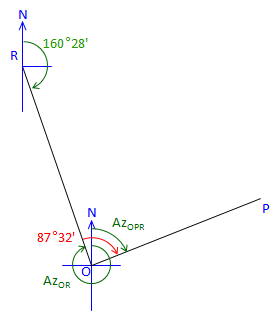## 3. Azimuth from Interior Angles

Given the following traverse and horizontal angles:Figure C-28 Azimuth Traverse

Using a azimuth of 68°00' for line OP, determine the azimuths of the remaining lines counter-clockwise around the traverse.

 At point P:Figure C-29 Line PQ is 92°48' right of Azimuth PO Az PO is the back azimuth of Az OP Az PQ = (Az OP + 180°00') + Angle PAz PQ = (68°00' + 180°00') + 92°48' = 340°48' At point Q:Figure C-30 Line QR is 112°26' to the right from Az QP Az QP is the back azimuth of Az PQ Az QR = (Az PQ + 180°00') + Angle QAz QR = (340°48'+180°00') + 112°26' = 633°14' Normalize: Az QR = 633°14' - 360°00' = 273°14' At point R:Figure C-31 Line RO is 67°14' right from Az RQ Az RQ is the back azimuth of Az QR Az RO = (Az QR + 180°00') + Angle RAz RO  = (273°14' + 180°00') + 67°14' = 520°28' Normalize: Az RO = 520°28' - 360°00' = 160°28' The directions for all four traverse lines have been computed. Angles at P, R, and R have been used, but not the angle at O. For a math check, use Azimuth RO and the angle at O to compute the original Az OP. At point O:Figure C-32 Line OP is 87°32' right of Az OR Az OR is the back azimuth of Az RO Az OP = (Az RO + 180°00') + Angle O Az OP = (160°28' + 180°00') + 87°32' = 428°00' Normalize: Az OP = 428°00' - 360°00' = 68°00' check!

Summary:

 Line Bearing OP 68°00' PQ 340°48' QR 273°14' RO 160°28'

There's a distinct pattern computing these azimuths:

New Az = (Previous Az + 180°00') + Angle.

This is true for a loop traverse meeting these conditions:

• Directions are counter-clockwise around the traverse, and,
• Angles are interior to the right.

What if the directions are clockwise around the traverse and interior angles counter-clockwise?

New Az = (Previous Az - 180°00') - Angle

Other patterns exist for clockwise travel with clockwise interior angles, clockwise exterior angles, counterclockwise exterior angles, etc. Rather than memorize the possible patterns, draw a sketch, and begin computing; the pattern will present itself after a few lines.